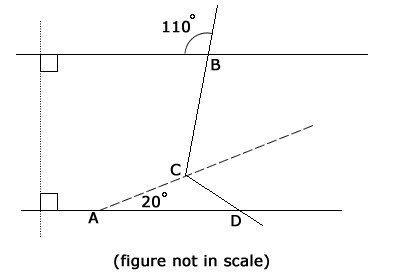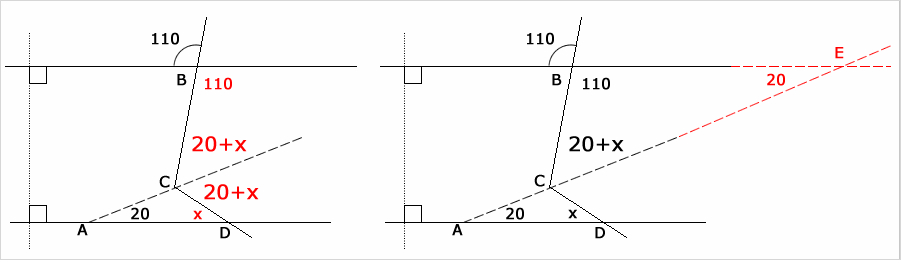GMAT Question of the Day: Daily via email | Daily via Instagram New to GMAT Club? Watch this Video

 It is currently 31 Mar 2020, 03:22### GMAT Club Daily Prep

#### Thank you for using the timer - this advanced tool can estimate your performance and suggest more practice questions. We have subscribed you to Daily Prep Questions via email.

Customized
for You

we will pick new questions that match your level based on your Timer History

Track

every week, we’ll send you an estimated GMAT score based on your performance

Practice
Pays

we will pick new questions that match your level based on your Timer History

#### Not interested in getting valuable practice questions and articles delivered to your email? No problem, unsubscribe here.# If line AC bisects angle BCD, what is the measure in d

Author Message
TAGS:

### Hide Tags

GMATH TeacherP
Status: GMATH founder
Joined: 12 Oct 2010
Posts: 936
If line AC bisects angle BCD, what is the measure in d  [#permalink]

### Show Tags

1
200:00

Difficulty:55% (hard)

Question Stats:45% (02:01) correct55% (03:12) wrongbased on 11 sessions

### HideShow timer Statistics

GMATH practice exercise (Quant Class 1)If line AC bisects angle BCD, what is the measure of angle ADC?

(A) 20 degrees
(B) 30 degrees
(C) 40 degrees
(D) 45 degrees
(E) 50 degrees

_________________
Fabio Skilnik :: GMATH method creator (Math for the GMAT)
Our high-level "quant" preparation starts here: https://gmath.net
InternB
Joined: 21 Apr 2014
Posts: 33
Re: If line AC bisects angle BCD, what is the measure in d  [#permalink]

### Show Tags

1
Lots of good lines/angles and triangles rules to refresh for this one:

-When two parallel lines are cut by a transversal, corresponding angles are equal
-Vertical angles are equal
-Supplemental angles sum to 180 degrees
-All the angles around one point sum to 360 degrees
-All three angles in a Triangle sum to 180 degrees

If you know all those rules and are comfortable with "extending lines," then this question isn't too challenging.AttachmentsIMG_2684.jpg [ 1.26 MiB | Viewed 413 times ]

_________________
Vivian Kerr | GMAT/GRE Tutor @ http://www.gmatrockstar.com | gmatrockstar[at]gmail.com | solid 5-star reviews on Yelp!

Former Kaplan and Grockit instructor. I've freelanced with Veritas Prep, Magoosh, and most of the bigger test prep companies. Now offering Skype-based private tutoring for the GMAT and GRE.

Reading Comprehension is my jam!GMATH TeacherP
Status: GMATH founder
Joined: 12 Oct 2010
Posts: 936
If line AC bisects angle BCD, what is the measure in d  [#permalink]

### Show Tags

fskilnik wrote:
GMATH practice exercise (Quant Class 1)If line AC bisects angle BCD, what is the measure of angle ADC?

(A) 20 degrees
(B) 30 degrees
(C) 40 degrees
(D) 45 degrees
(E) 50 degreesAll angles are measured in degrees.

$$? = x$$

$$\left( 1 \right)\,\,{\text{angle}}\,\,DCE\,\,\, = \,\,\,20 + x\,\,\,\,\,\,\left[ {\,{\text{exterior}}\,\,{\text{angle}}\,\,{\text{of}}\,\,\Delta ADC\,} \right]$$

$$\left( 2 \right)\,\,{\text{angle}}\,\,ECB\,\,\, = \,\,\,20 + x\,\,\,\,\,\,\left[ {\,{\text{line}}\,\,AC\,\,{\text{bisects}}\,\,\,{\text{angle}}\,\,BCD\,} \right]$$

$$\left( 3 \right)\,\,{\text{angle}}\,\,DAC\,\,\, = \,\,\,{\text{angle}}\,\,AEB\,\,\, = \,\,\,20\,\,\,\,\,\,\left[ {\,{\text{lines}}\,\,AD\,\,{\text{and}}\,\,BE\,\,{\text{are}}\,\,{\text{parallel}}\,} \right]$$

$$\Delta BCE\,\,:\,\,\,20 + x = 180 - \left( {110 + 20} \right)\,\,\,\,\, \Rightarrow \,\,\,\,\,? = x = 30$$

We follow the notations and rationale taught in the GMATH method.

Regards,
Fabio.
_________________
Fabio Skilnik :: GMATH method creator (Math for the GMAT)
Our high-level "quant" preparation starts here: https://gmath.netIf line AC bisects angle BCD, what is the measure in d   [#permalink] 03 Mar 2019, 14:00
Display posts from previous: Sort by

# If line AC bisects angle BCD, what is the measure in d